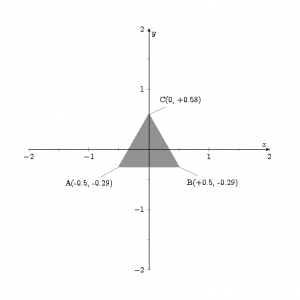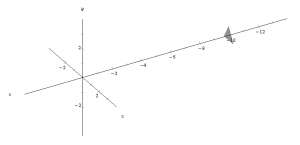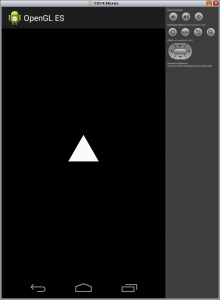Categories

# Drawing a triangle with OpenGL ES in Android

The scope of this post is drawing a triangle with OpenGL ES 1.x in Android focusing especially on the relationship between code and position of the triangle.
The coordinate system has the origin where the observer is, with the x axis horizontal and rightward, the y-axis vertical and upward and the z axis points so that the observer is looking towards the negative z, then the coordinate system follows the right-hand rule.
The 3 vertices of the triangle have coordinates:

• A(-0.5, -0.29, -10)
• B(+0.5, -0.29, -10)
• C(0, +0.58, -10)

that are the vertices of an equilateral triangle with the barycenter in (0, 0, -10).

In our view the triangle appears as in figure:If we go out from our perspective the scenario appears as in the figure below where the observer is located at the origin of the axesTo draw the triangle with OpenGL ES 1.x in Android follow these steps:

1. create an Android project without initial activity
2. edit the file AndroidManifest.xml
```<manifest
xmlns:android="http://schemas.android.com/apk/res/android"
package="eu.lucazanini.opengl.shape"
android:versionCode="1"
android:versionName="1.0">

<uses-sdk
android:minSdkVersion="5"
android:targetSdkVersion="17" />

<application
android:icon="@drawable/ic_launcher"
android:label="@string/app_name"
android:theme="@style/AppTheme"
android:allowBackup="true">
<activity
android:name=".MainActivity"
android:label="@string/app_name">
<intent-filter>
<action android:name="android.intent.action.MAIN" />
<category android:name="android.intent.category.LAUNCHER" />
</intent-filter>
</activity>
</application>

</manifest>
```
3. create the class eu/lucazanini/opengl/shape/MainActivity.java
```package eu.lucazanini.opengl.shape;

import android.app.Activity;
import android.opengl.GLSurfaceView;
import android.os.Bundle;
import android.view.WindowManager;

public class MainActivity extends Activity {

GLSurfaceView view;

@Override
public void onCreate(Bundle savedInstanceState) {
super.onCreate(savedInstanceState);

getWindow().setFlags(WindowManager.LayoutParams.FLAG_FULLSCREEN, WindowManager.LayoutParams.FLAG_FULLSCREEN);
view = new GLSurfaceView(this);
view.setRenderer(new ShapeRenderer());
setContentView(view);
}

@Override
protected void onPause() {
super.onPause();
view.onPause();
}

@Override
protected void onResume() {
super.onResume();
view.onResume();
}

}
```

in the onCreate event:

• row 16: I set the full screen
• row 17: I create an object GLSurfaceView
• row 18: I call the method GLSurfaceView.setRenderer passing as argument a object GLSurfaceView.Renderer
• row 19: I set the instance just created of GLSurfaceView as view of the Activity calling the method setContentView

in the onResume and onPause methods I notify the same events to GLSurfaceView

4. create the class eu/lucazanini/opengl/shape/ShapeRenderer.java
```package eu.lucazanini.opengl.shape;

import javax.microedition.khronos.egl.EGLConfig;
import javax.microedition.khronos.opengles.GL10;
import javax.microedition.khronos.opengles.GL11;

import android.opengl.GLSurfaceView;

public class ShapeRenderer implements GLSurfaceView.Renderer {

private Triangle shape;

public ShapeRenderer() {
shape = new Triangle();
}

@Override
public void onDrawFrame(GL10 gl) {

gl.glClear(GL11.GL_COLOR_BUFFER_BIT | GL11.GL_DEPTH_BUFFER_BIT);

gl.glMatrixMode(GL11.GL_MODELVIEW);

// gl.glTranslatef(1.0f, 0.0f, 0.0f);
// gl.glRotatef(90.0f, 0.0f, 0.0f, 1.0f);

gl.glEnableClientState(GL11.GL_VERTEX_ARRAY);

shape.draw(gl);

gl.glDisableClientState(GL11.GL_VERTEX_ARRAY);
}

@Override
public void onSurfaceChanged(GL10 gl, int width, int height) {

gl.glViewport(0, 0, width, height);

float ratio;
float zNear = .1f;
float zFar = 1000f;
float fieldOfView = (float) Math.toRadians(30);
float size;

gl.glEnable(GL11.GL_NORMALIZE);

ratio = (float) width / (float) height;

gl.glMatrixMode(GL11.GL_PROJECTION);

size = zNear * (float) (Math.tan((double) (fieldOfView / 2.0f)));

gl.glFrustumf(-size, size, -size / ratio, size / ratio, zNear, zFar);

gl.glMatrixMode(GL11.GL_MODELVIEW);
}

@Override
public void onSurfaceCreated(GL10 gl, EGLConfig config) {

gl.glDisable(GL11.GL_DITHER);

gl.glHint(GL11.GL_PERSPECTIVE_CORRECTION_HINT, GL11.GL_FASTEST);

gl.glClearColor(0, 0, 0, 0);

gl.glEnable(GL11.GL_CULL_FACE);
gl.glFrontFace(GL11.GL_CCW);

gl.glEnable(GL11.GL_DEPTH_TEST);
}

}
```

this class implements 3 methods of GLSurfaceView.Renderer

• onSurfaceCreated method: it is called only one time when GLSurfaceView is created, and it sets the openGL environment, especially:
• row 66: it draws a black background
• row 68: it enables the option to show or hide the triangle depending on whether the vertices are ordered clockwise or counterclockwise; this instruction works together with gl.glFrontFace (GL11.GL_CCW); in the next row
• onDrawFrame method: it is called every time you need to draw GLSurfaceView; note the call to draw method of the Triangle class
• onSurfaceChanged method: it is called when the dimensions of GLSurfaceView change, here I define the frustrum, zNear and ZFar are the distance from the minor base (near plane) and major base (far plane) and fieldView is the visual field measured in radians (in the example it is 0.52 rad or 30°); the object is shown ony if it is inside the frustrum
5. create the class eu/lucazanini/opengl/shape/Triangle.java
```package eu.lucazanini.opengl.shape;

import java.nio.ByteBuffer;
import java.nio.ByteOrder;
import java.nio.FloatBuffer;

import javax.microedition.khronos.opengles.GL10;
import javax.microedition.khronos.opengles.GL11;

public class Triangle {

private FloatBuffer mFVertexBuffer;
private ByteBuffer mIndexBuffer;

public Triangle() {

float vertices[] = {
-0.5f, -0.29f, -10f,
0.5f, -0.29f, -10f,
0f, 0.58f, -10f
};

byte indices[] = { 0, 1, 2 };

mFVertexBuffer = makeFloatBuffer(vertices);

mIndexBuffer = ByteBuffer.allocateDirect(indices.length);
mIndexBuffer.put(indices);
mIndexBuffer.position(0);
}

public void draw(GL10 gl) {
gl.glVertexPointer(3, GL11.GL_FLOAT, 0, mFVertexBuffer);
gl.glDrawElements(GL11.GL_TRIANGLES, 3, GL11.GL_UNSIGNED_BYTE, mIndexBuffer);
}

private static FloatBuffer makeFloatBuffer(float[] arr) {
ByteBuffer bb = ByteBuffer.allocateDirect(arr.length * 4);
bb.order(ByteOrder.nativeOrder());
FloatBuffer fb = bb.asFloatBuffer();
fb.put(arr);
fb.position(0);
return fb;
}
}
```
• row 17: the variable vertices contains the coordinates (x, y, z) of the 3 vertices of the triangle
• row 23: the variable indices sorts the vertices counterclockwise
• row 32: the method draw draws the triangle
• row 33: glVertexPointer defines an array containing the vertices
• row 34: glDrawElements renders primitives from array data
6. launch the applicationYou can test different combinations of zNear, Zfar and fieldView for the frustrum but remember that you don’t see the triangle if it is outside the frustrum.
You can uncomment the rows 25 and 26 of the class ShapeRenderer and verify how you can translate the triangle with glTranslatef or how you can rotate it with glRotatef; using glRotatef if the rotation means that the vertices of the triangle are sorted counterclockwise for the observer, the triangle will not displayed.

## 2 replies on “Drawing a triangle with OpenGL ES in Android”Saeed Aslamisays:

Tanks very much is good…..!

[…] the post Drawing a triangle with OpenGL ES in Android I explained how to draw a triangle without setting the colors, indeed, the triangle is gray. In […]

This site uses Akismet to reduce spam. Learn how your comment data is processed.